Documentation

# EstimatedInfo object

Object containing information about estimated model quantities

## Description

The `estimatedInfo` object contains information about estimated model quantities (species, parameters, or compartments). Use this object to specify which quantities in a SimBiology® model are estimated, what parameter transforms are used, and optionally, the initial estimates for these quantities. Use this object to specify what quantities in a SimBiology model are estimated when using `sbiofit` or `sbiofitmixed`.

## Construction

`estimInfo = estimatedInfo` creates an empty `estimatedInfo` object.

`estimInfoArray = estimatedInfo(transformedNames)` creates an array of `estimatedInfo` objects for quantities specified in `transformedNames`. The initial values for these quantities are obtained from the SimBiology model when you run `sbiofit` or `sbiofitmixed`.

`estimInfoArray = estimatedInfo(___,'InitialTransformedValue',itValues)` defines the initial transformed values of model quantities specified by `itValues`. You cannot specify this name-value pair along with the `'InitialValue'` name-value pair.

`estimInfoArray = estimatedInfo(___,'InitialValue',iValues)` defines the initial values of model quantities specified by `iValues`. You cannot specify this name-value pair along with the `'InitialTransformedValue'` name-value pair.

`estimInfoArray = estimatedInfo(___,'Bounds',boundValues)` defines the lower and upper bounds for parameter estimation specified by `boundValues`. You cannot specify this name-value pair along with the `'TransformedBounds'` name-value pair. All methods support parameter bounds in `sbiofit` (that is, `fminsearch`, `nlinfit`, `fminunc`, `fmincon`, `lsqcurvefit`, `lsqnonlin`, `patternsearch`, `ga`, `particleswarm`, and scattersearch). When using `fminsearch`, `nlinfit`, or `fminunc` with bounds, the objective function returns `Inf` if bounds are exceeded. When you turn on options such as `FunValCheck`, the optimization may error if bounds are exceeded during estimation. If using `nlinfit`, it may report warnings about the Jacobian being ill-conditioned or not being able to estimate if the final result is too close to the bounds. `sbiofitmixed` does not support parameter bounds.

`estimInfoArray = estimatedInfo(___,'TransformedBounds',tBoundValues)` defines the transformed bounds for parameter estimation specified by `tBoundValues`. You cannot specify this name-value pair along with the `'Bounds'` name-value pair.

`estimInfoArray = estimatedInfo(___,'CategoryVariableName',groups)` defines which groups in the data have separate estimated values for parameters. In other words, this allows you to estimate parameter values specific for each group or category. For example, you can estimate parameters based on individuals’ age or sex.

## Input Arguments

collapse all

Names of estimated model quantities, specified as a character vector, string, string vector, or cell array of character vectors. Each character vector (or string) must be in one of these formats:

• Name or qualified name of a model quantity, such as `'Cl'`, `'Reaction1.k'`,```'[c 1].[r 1]'```

• Name of a supported parameter transform (`log`, `logit`, or `probit`) followed by a quantity name in parentheses, such as `'log(Cl)'`, `'logit(Reaction1.k)'`, `'probit([c 1].[r 1])'`

Initial transformed values of model quantities, specified as a vector or cell array. It must have the same length as `transformedNames`. If it is a cell array, each element of the cell must be a scalar or the empty matrix `[]`.

Initial values of model quantities, specified as a vector or cell array. It must have the same length as `transformedNames`. If it is a cell array, each element of the cell must be a scalar or the empty matrix `[]`.

Bound constraints for estimated parameters, specified as a matrix or cell array. If `boundValues` is a matrix, it is a N-by-2 matrix of numbers, where N is either 1 or the length of `transformedNames`. If it is a cell array, each element must be a vector of size 1-by-2 or empty `[]`.

Each row of `boundValues` corresponds to the lower (the first number) and upper (the second number) bounds of each element (such as a parameter) of `estimInfo`. The lower bound must be less than the upper bound. If you specify a single row, these bounds are applied to all elements of `estimInfoArray`.

You cannot specify this name-value pair along with the `'TransformedBounds'` name-value pair.

Transformed bound constraints for estimated parameters, specified as a matrix or cell array. `tBoundValues` is a N-by-2 matrix of numbers, where N is either 1 or the length of `transformedNames`. If it is a cell array, each element must be a vector of size 1-by-2 or empty `[]`.

Each row of `tBoundValues` corresponds to the lower (the first number) and upper (the second number) bounds of each element (such as a parameter) of `estimInfo`. The lower bound must be less than the upper bound. If you specify a single row, the bounds are applied to all elements of `estimInfoArray`.

You cannot specify this name-value pair along with the `'Bounds'` name-value pair.

Group names for estimated parameters, specified as a character vector, string, string vector, or cell array of character vectors. Each character vector (or string) must be one of the following.

• Name of a variable in the data used for fitting

• `'<GroupVariableName>'` (default)

• `'<None>'`

`'<GroupVariableName>'` indicates that each group in the data uses a separate parameter estimate. `'<None>'` indicates that all groups in the data use the same parameter estimate.

If the data you plan to use for fitting contains variables that group data into different categories, you can specify the names of those variables. For instance, if you have a variable called `Sex` which indicates male and female individuals, you can specify `'Sex'` as the `'CategoryVariableName'`. This means that all male individuals have one set of parameter estimates and all females have a separate set.

## Output Arguments

collapse all

Estimated model quantity, returned as an `estimatedInfo` object.

Estimated model quantities, returned as an `estimatedInfo` object or vector of `estimatedInfo` objects. If `transformedNames` is a single character vector, `estimInfoArray` is a scalar `estimatedInfo` object. Otherwise, `estimInfoArray` is a vector of `estimatedInfo` objects with the same length as the input argument `transformedNames`.

## Properties

 `Name` Character vector indicating the name of an estimated model quantity. Changing this property also updates the `TransformedName` property. `Transform` Character vector indicating whether the quantity value is transformed during estimation. Valid names are `''`, `'log'`, `'logit'`, and `'probit'`. An empty character vector `''` indicates that no transform is applied.A log transform ensures that the component value is always positive during estimation. The `logit` and `probit` transforms constrain component values to lie between 0 and 1.The `probit` function is the inverse cumulative distribution function associated with the standard normal distribution. For the `probit` transform, SimBiology uses the `norminv` function. Hence Statistics and Machine Learning Toolbox™ is required for the transform.The logit function, which is the inverse of sigmoid function, is defined as ```logit(x) = log(x) – log(1 – x)```. `TransformedName` Read-only character vector that combines the transform name (such as `'log'`) and the quantity name (such as `'Central'`) into an expression (such as `'log(Central)'`). `InitialValue` Empty matrix `[]` or real, finite, scalar value specifying the initial values of model quantities used for estimation. The empty matrix indicates that the initial values for estimation are obtained from the relevant quantity property (`Value` for parameters, `InitialAmount` for species, and `Capacity` for compartments).Changing this property automatically updates the `InitialTransformedValue` property of corresponding model quantities. `InitialTransformedValue` Empty matrix `[]` or scalar value specifying the initial transformed values of model quantities used for estimation. The empty matrix indicates that the initial transformed values for estimation are obtained by transforming the relevant quantity property (`Value` for parameters, `InitialAmount` for species, and `Capacity` for compartments).Changing this property automatically updates the `InitialValue` property of corresponding model quantities. `Bounds` Empty matrix `[]` or 1-by-2 vector of real, finite value specifying the lower and upper bound for an estimated parameter. The empty matrix `[]` indicates that the only bound constraints are those introduced by the value of `Transform`. For example, setting `Transform` to `'log'` constrains the parameter to the range `[0,inf]`. Changing this property also updates `TransformedBounds`.The lower bound must be less than the upper bound. `TransformedBounds` Empty matrix `[]` or 1-by-2 vector of real, finite value specifying the lower and upper bound for an estimated parameter. The empty matrix `[]` indicates that the value of the parameter in transformed space is not constrained. Changing this property also updates `Bounds`.The lower bound must be less than the upper bound. `CategoryVariableName` Character vector or cell array of character vectors specifying which groups in the data have separate estimated values for the parameter. The character vector can be the name of a variable in the data used for fitting or one of the keywords: `''` or `''`.`''` indicates that each group in the data uses a separate parameter estimate. `''` indicates that all groups in the data use the same parameter estimate.If you specify `'Pooled'` name-value pair argument (to either `true` or `false`) when you run `sbiofit`, then the function ignores this variable. `sbiofitmixed` always ignores this property.

## Examples

collapse all

Create a one-compartment PK model with bolus dosing and linear clearance.

```pkmd = PKModelDesign; pkc1 = addCompartment(pkmd,'Central'); pkc1.DosingType = 'Bolus'; pkc1.EliminationType = 'linear-clearance'; pkc1.HasResponseVariable = true;```

Suppose you want to estimate the volume of the central compartment (`Central`). You can specify such estimated model quantity as well as appropriate parameter transform (`log` transform in this example), initial value, and parameter bound using the `estimatedInfo` object.

`estimated = estimatedInfo('log(Central)','InitialValue', 1,'Bounds',[0 10])`
```estimated = estimatedInfo with properties: Name: 'Central' Transform: 'log' TransformedName: 'log(Central)' InitialValue: 1 InitialTransformedValue: 0 Bounds: [0 10] TransformedBounds: [-Inf 2.3026] CategoryVariableName: '<GroupVariableName>' ```

Background

This example shows how to fit an individual's PK profile data to one-compartment model and estimate pharmacokinetic parameters.

Suppose you have drug plasma concentration data from an individual and want to estimate the volume of the central compartment and the clearance. Assume the drug concentration versus the time profile follows the monoexponential decline ${C}_{t}={C}_{0}{e}^{-{k}_{e}t}$, where ${C}_{t}$ is the drug concentration at time t, ${C}_{0}$ is the initial concentration, and ${k}_{e}$ is the elimination rate constant that depends on the clearance and volume of the central compartment ${k}_{e}=Cl/V$.

The synthetic data in this example was generated using the following model and parameters:

• One-compartment model with bolus dosing and first-order elimination

• Volume of the central compartment (`Central`) = 1.70 liter

• Clearance parameter (`Cl_Central`) = 0.55 liter/hour

• Constant error model

The data is stored as a table with variables `Time` and `Conc` that represent the time course of the plasma concentration of an individual after an intravenous bolus administration measured at 13 different time points. The variable units for `Time` and `Conc` are hour and milligram/liter, respectively.

```clear all load(fullfile(matlabroot,'examples','simbio','data15.mat'))```
```plot(data.Time,data.Conc,'b+') xlabel('Time (hour)'); ylabel('Drug Concentration (milligram/liter)');```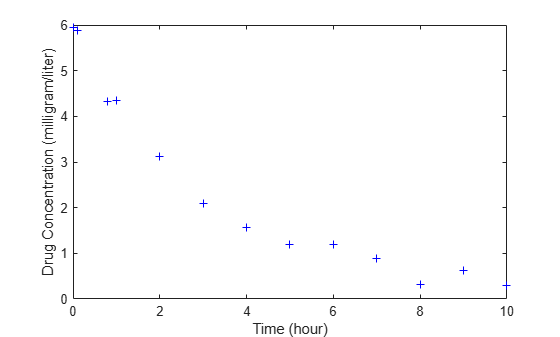Convert to groupedData Format

Convert the data set to a `groupedData` object, which is the required data format for the fitting function `sbiofit` for later use. A `groupedData` object also lets you set independent variable and group variable names (if they exist). Set the units of the `Time` and `Conc` variables. The units are optional and only required for the `UnitConversion` feature, which automatically converts matching physical quantities to one consistent unit system.

```gData = groupedData(data); gData.Properties.VariableUnits = {'hour','milligram/liter'}; gData.Properties```
```ans = struct with fields: Description: '' UserData: [] DimensionNames: {'Row' 'Variables'} VariableNames: {'Time' 'Conc'} VariableDescriptions: {} VariableUnits: {'hour' 'milligram/liter'} VariableContinuity: [] RowNames: {} CustomProperties: [1x1 matlab.tabular.CustomProperties] GroupVariableName: '' IndependentVariableName: 'Time' ```

`groupedData` automatically set the name of the `IndependentVariableName` property to the `Time` variable of the data.

Construct a One-Compartment Model

Use the built-in PK library to construct a one-compartment model with bolus dosing and first-order elimination where the elimination rate depends on the clearance and volume of the central compartment. Use the `configset` object to turn on unit conversion.

```pkmd = PKModelDesign; pkc1 = addCompartment(pkmd,'Central'); pkc1.DosingType = 'Bolus'; pkc1.EliminationType = 'linear-clearance'; pkc1.HasResponseVariable = true; model = construct(pkmd); configset = getconfigset(model); configset.CompileOptions.UnitConversion = true;```

For details on creating compartmental PK models using the SimBiology® built-in library, see Create Pharmacokinetic Models.

Define Dosing

Define a single bolus dose of 10 milligram given at time = 0. For details on setting up different dosing schedules, see Doses in SimBiology Models.

```dose = sbiodose('dose'); dose.TargetName = 'Drug_Central'; dose.StartTime = 0; dose.Amount = 10; dose.AmountUnits = 'milligram'; dose.TimeUnits = 'hour';```

Map Response Data to the Corresponding Model Component

The data contains drug concentration data stored in the `Conc` variable. This data corresponds to the `Drug_Central` species in the model. Therefore, map the data to `Drug_Central` as follows.

`responseMap = {'Drug_Central = Conc'};`

Specify Parameters to Estimate

The parameters to fit in this model are the volume of the central compartment (Central) and the clearance rate (Cl_Central). In this case, specify log-transformation for these biological parameters since they are constrained to be positive. The `estimatedInfo` object lets you specify parameter transforms, initial values, and parameter bounds if needed.

```paramsToEstimate = {'log(Central)','log(Cl_Central)'}; estimatedParams = estimatedInfo(paramsToEstimate,'InitialValue',[1 1],'Bounds',[1 5;0.5 2]);```

Estimate Parameters

Now that you have defined one-compartment model, data to fit, mapped response data, parameters to estimate, and dosing, use `sbiofit` to estimate parameters. The default estimation function that `sbiofit` uses will change depending on which toolboxes are available. To see which function was used during fitting, check the `EstimationFunction` property of the corresponding results object.

`fitConst = sbiofit(model,gData,responseMap,estimatedParams,dose);`

Display Estimated Parameters and Plot Results

Notice the parameter estimates were not far off from the true values (1.70 and 0.55) that were used to generate the data. You may also try different error models to see if they could further improve the parameter estimates.

`fitConst.ParameterEstimates`
```ans=2×4 table Name Estimate StandardError Bounds ______________ ________ _____________ __________ {'Central' } 1.6993 0.034821 1 5 {'Cl_Central'} 0.53358 0.01968 0.5 2 ```
```s.Labels.XLabel = 'Time (hour)'; s.Labels.YLabel = 'Concentration (milligram/liter)'; plot(fitConst,'AxesStyle',s);```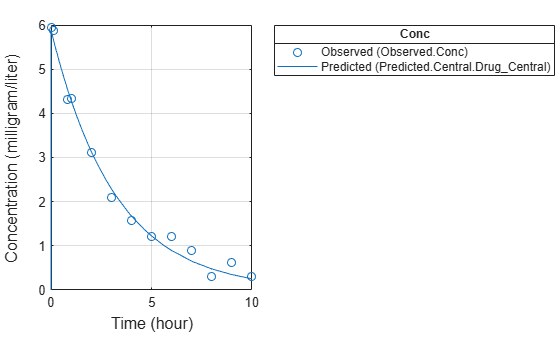Use Different Error Models

Try three other supported error models (proportional, combination of constant and proportional error models, and exponential).

```fitProp = sbiofit(model,gData,responseMap,estimatedParams,dose,... 'ErrorModel','proportional'); fitExp = sbiofit(model,gData,responseMap,estimatedParams,dose,... 'ErrorModel','exponential'); fitComb = sbiofit(model,gData,responseMap,estimatedParams,dose,... 'ErrorModel','combined');```

Use Weights Instead of an Error Model

You can specify weights as a numeric matrix, where the number of columns corresponds to the number of responses. Setting all weights to 1 is equivalent to the constant error model.

```weightsNumeric = ones(size(gData.Conc)); fitWeightsNumeric = sbiofit(model,gData,responseMap,estimatedParams,dose,'Weights',weightsNumeric);```

Alternatively, you can use a function handle that accepts a vector of predicted response values and returns a vector of weights. In this example, use a function handle that is equivalent to the proportional error model.

```weightsFunction = @(y) 1./y.^2; fitWeightsFunction = sbiofit(model,gData,responseMap,estimatedParams,dose,'Weights',weightsFunction);```

Compare Information Criteria for Model Selection

Compare the loglikelihood, AIC, and BIC values of each model to see which error model best fits the data. A larger likelihood value indicates the corresponding model fits the model better. For AIC and BIC, the smaller values are better.

```allResults = [fitConst,fitWeightsNumeric,fitWeightsFunction,fitProp,fitExp,fitComb]; errorModelNames = {'constant error model','equal weights','proportional weights', ... 'proportional error model','exponential error model',... 'combined error model'}; LogLikelihood = [allResults.LogLikelihood]'; AIC = [allResults.AIC]'; BIC = [allResults.BIC]'; t = table(LogLikelihood,AIC,BIC); t.Properties.RowNames = errorModelNames; t```
```t=6×3 table LogLikelihood AIC BIC _____________ _______ _______ constant error model 3.9866 -3.9732 -2.8433 equal weights 3.9866 -3.9732 -2.8433 proportional weights -3.8472 11.694 12.824 proportional error model -3.8257 11.651 12.781 exponential error model 1.1984 1.6032 2.7331 combined error model 3.9163 -3.8326 -2.7027 ```

Based on the information criteria, the constant error model (or equal weights) fits the data best since it has the largest loglikelihood value and the smallest AIC and BIC.

Display Estimated Parameter Values

Show the estimated parameter values of each model.

```Estimated_Central = zeros(6,1); Estimated_Cl_Central = zeros(6,1); t2 = table(Estimated_Central,Estimated_Cl_Central); t2.Properties.RowNames = errorModelNames; for i = 1:height(t2) t2{i,1} = allResults(i).ParameterEstimates.Estimate(1); t2{i,2} = allResults(i).ParameterEstimates.Estimate(2); end t2```
```t2=6×2 table Estimated_Central Estimated_Cl_Central _________________ ____________________ constant error model 1.6993 0.53358 equal weights 1.6993 0.53358 proportional weights 1.9045 0.51734 proportional error model 1.8777 0.51147 exponential error model 1.7872 0.51701 combined error model 1.7008 0.53271 ```

Conclusion

This example showed how to estimate PK parameters, namely the volume of the central compartment and clearance parameter of an individual, by fitting the PK profile data to one-compartment model. You compared the information criteria of each model and estimated parameter values of different error models to see which model best explained the data. Final fitted results suggested both the constant and combined error models provided the closest estimates to the parameter values used to generate the data. However, the constant error model is a better model as indicated by the loglikelihood, AIC, and BIC information criteria.

This example shows how to estimate category-specific (such as young versus old, male versus female), individual-specific, and population-wide parameters using PK profile data from multiple individuals.

Background

Suppose you have drug plasma concentration data from 30 individuals and want to estimate pharmacokinetic parameters, namely the volumes of central and peripheral compartment, the clearance, and intercompartmental clearance. Assume the drug concentration versus the time profile follows the biexponential decline ${C}_{t}=A{e}^{-at}+B{e}^{-bt}$, where ${C}_{t}$ is the drug concentration at time t, and $a$ and $b$ are slopes for corresponding exponential declines.

This synthetic data contains the time course of plasma concentrations of 30 individuals after a bolus dose (100 mg) measured at different times for both central and peripheral compartments. It also contains categorical variables, namely `Sex` and `Age`.

```clear load(fullfile(matlabroot,'examples','simbio','sd5_302RAgeSex.mat'))```

Convert to groupedData Format

Convert the data set to a `groupedData` object, which is the required data format for the fitting function `sbiofit`. A `groupedData` object also allows you set independent variable and group variable names (if they exist). Set the units of the `ID`, `Time`, `CentralConc`, `PeripheralConc`, `Age`, and `Sex` variables. The units are optional and only required for the `UnitConversion` feature, which automatically converts matching physical quantities to one consistent unit system.

```gData = groupedData(data); gData.Properties.VariableUnits = {'','hour','milligram/liter','milligram/liter','',''}; gData.Properties```
```ans = struct with fields: Description: '' UserData: [] DimensionNames: {'Row' 'Variables'} VariableNames: {1x6 cell} VariableDescriptions: {} VariableUnits: {1x6 cell} VariableContinuity: [] RowNames: {} CustomProperties: [1x1 matlab.tabular.CustomProperties] GroupVariableName: 'ID' IndependentVariableName: 'Time' ```

The `IndependentVariableName` and `GroupVariableName` properties have been automatically set to the `Time` and `ID` variables of the data.

Visualize Data

Display the response data for each individual.

```t = sbiotrellis(gData,'ID','Time',{'CentralConc','PeripheralConc'},... 'Marker','+','LineStyle','none'); % Resize the figure. t.hFig.Position(:) = [100 100 1280 800];```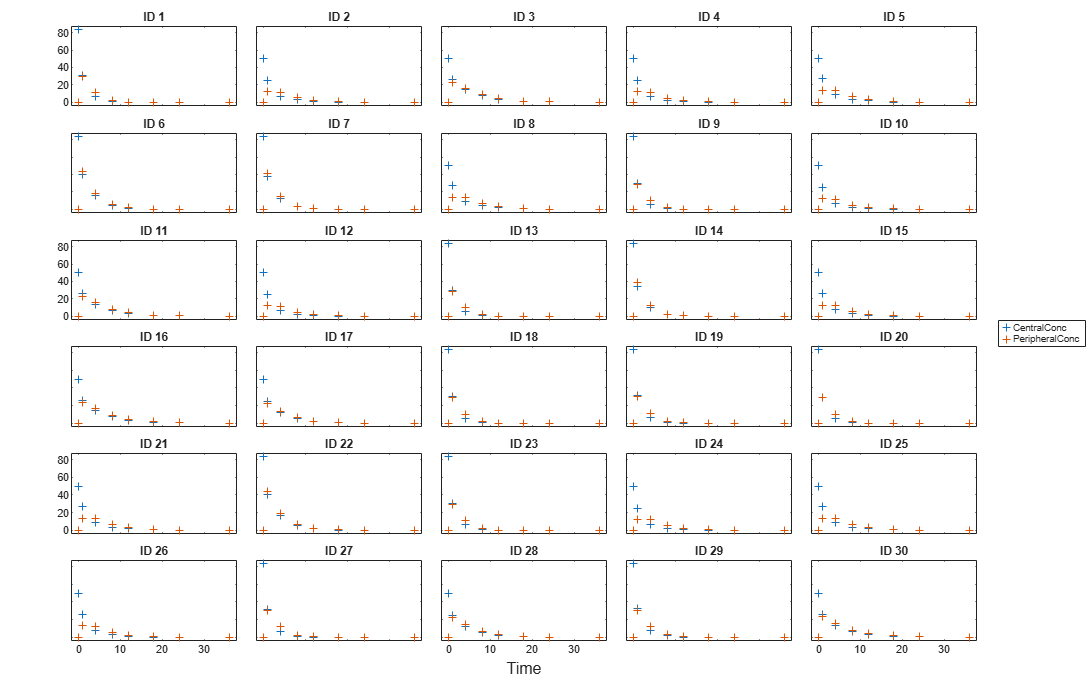Set Up a Two-Compartment Model

Use the built-in PK library to construct a two-compartment model with infusion dosing and first-order elimination where the elimination rate depends on the clearance and volume of the central compartment. Use the `configset` object to turn on unit conversion.

```pkmd = PKModelDesign; pkc1 = addCompartment(pkmd,'Central'); pkc1.DosingType = 'Bolus'; pkc1.EliminationType = 'linear-clearance'; pkc1.HasResponseVariable = true; pkc2 = addCompartment(pkmd,'Peripheral'); model = construct(pkmd); configset = getconfigset(model); configset.CompileOptions.UnitConversion = true;```

For details on creating compartmental PK models using the SimBiology® built-in library, see Create Pharmacokinetic Models.

Define Dosing

Assume every individual receives a bolus dose of 100 mg at time = 0. For details on setting up different dosing strategies, see Doses in SimBiology Models.

```dose = sbiodose('dose','TargetName','Drug_Central'); dose.StartTime = 0; dose.Amount = 100; dose.AmountUnits = 'milligram'; dose.TimeUnits = 'hour';```

Map the Response Data to Corresponding Model Components

The data contains measured plasma concentration in the central and peripheral compartments. Map these variables to the appropriate model components, which are `Drug_Central` and `Drug_Peripheral`.

`responseMap = {'Drug_Central = CentralConc','Drug_Peripheral = PeripheralConc'};`

Specify Parameters to Estimate

Specify the volumes of central and peripheral compartments `Central` and `Peripheral`, intercompartmental clearance `Q12`, and clearance `Cl_Central` as parameters to estimate. The `estimatedInfo` object lets you optionally specify parameter transforms, initial values, and parameter bounds. Since both `Central` and `Peripheral` are constrained to be positive, specify a log-transform for each parameter.

```paramsToEstimate = {'log(Central)', 'log(Peripheral)', 'Q12', 'Cl_Central'}; estimatedParam = estimatedInfo(paramsToEstimate,'InitialValue',[1 1 1 1]);```

Estimate Individual-Specific Parameters

Estimate one set of parameters for each individual by setting the `'Pooled'` name-value pair argument to `false`.

`unpooledFit = sbiofit(model,gData,responseMap,estimatedParam,dose,'Pooled',false);`

Display Results

Plot the fitted results versus the original data for each individual (group).

```t = plot(unpooledFit); % Resize the figure. t.hFig.Position(:) = [100 100 1280 800];```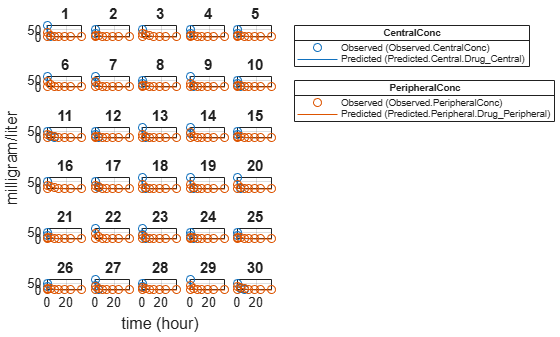For an unpooled fit, `sbiofit` always returns one results object for each individual.

Examine Parameter Estimates for Category Dependencies

Explore the unpooled estimates to see if there is any category-specific parameters, that is, if some parameters are related to one or more categories. If there are any category dependencies, it might be possible to reduce the number of degrees of freedom by estimating just category-specific values for those parameters.

First extract the ID and category values for each ID

`catParamValues = unique(gData(:,{'ID','Sex','Age'}));`

Add variables to the table containing each parameter's estimate.

```allParamValues = vertcat(unpooledFit.ParameterEstimates); catParamValues.Central = allParamValues.Estimate(strcmp(allParamValues.Name, 'Central')); catParamValues.Peripheral = allParamValues.Estimate(strcmp(allParamValues.Name, 'Peripheral')); catParamValues.Q12 = allParamValues.Estimate(strcmp(allParamValues.Name, 'Q12')); catParamValues.Cl_Central = allParamValues.Estimate(strcmp(allParamValues.Name, 'Cl_Central'));```

Plot estimates of each parameter for each category. `gscatter` requires Statistics and Machine Learning Toolbox™. If you do not have it, use other alternative plotting functions such as `plot`.

```h = figure; ylabels = {'Central','Peripheral','Cl\_Central','Q12'}; plotNumber = 1; for i = 1:4 thisParam = estimatedParam(i).Name; % Plot for Sex category subplot(4,2,plotNumber); plotNumber = plotNumber + 1; gscatter(double(catParamValues.Sex), catParamValues.(thisParam), catParamValues.Sex); ax = gca; ax.XTick = []; ylabel(ylabels(i)); legend('Location','bestoutside') % Plot for Age category subplot(4,2,plotNumber); plotNumber = plotNumber + 1; gscatter(double(catParamValues.Age), catParamValues.(thisParam), catParamValues.Age); ax = gca; ax.XTick = []; ylabel(ylabels(i)); legend('Location','bestoutside') end % Resize the figure. h.Position(:) = [100 100 1280 800];```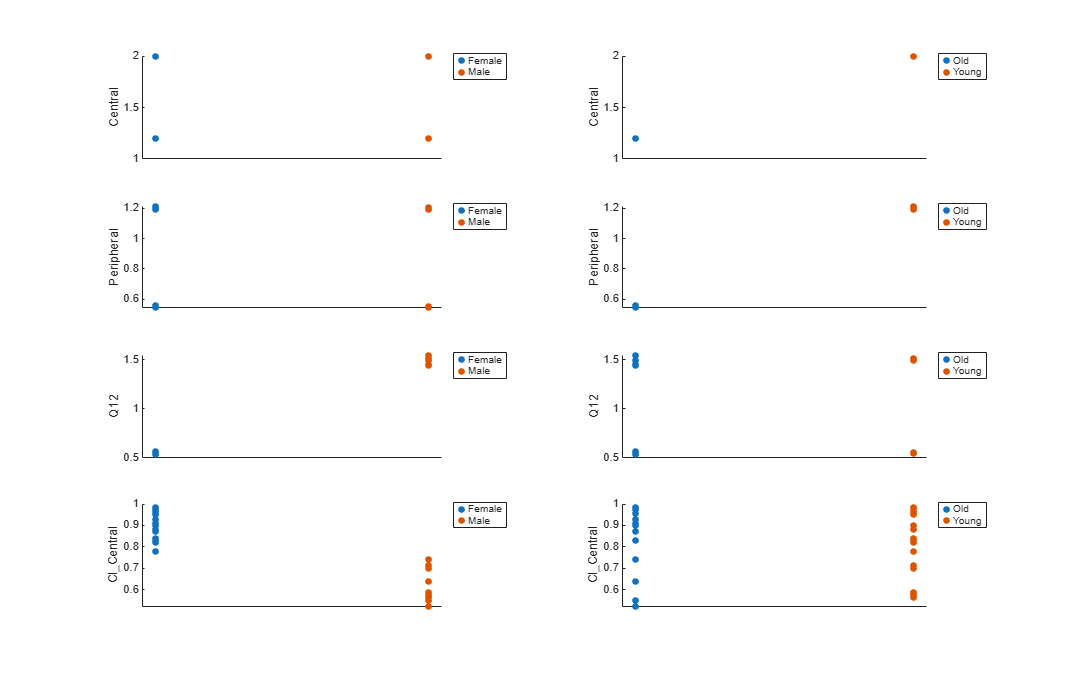Based on the plot, it seems that young individuals tend to have higher volumes of central and peripheral compartments (`Central`, `Peripheral`) than old individuals (that is, the volumes seem to be age-specific). In addition, males tend to have higher clearance rates (`Cl_Central`) than females but the opposite for the Q12 parameter (that is, the clearance and Q12 seem to be sex-specific).

Estimate Category-Specific Parameters

Use the `'CategoryVariableName'` property of the `estimatedInfo` object to specify which category to use during fitting. Use `'Sex'` as the group to fit for the clearance `Cl_Central` and `Q12` parameters. Use `'Age'` as the group to fit for the `Central` and `Peripheral` parameters.

```estimatedParam(1).CategoryVariableName = 'Age'; estimatedParam(2).CategoryVariableName = 'Age'; estimatedParam(3).CategoryVariableName = 'Sex'; estimatedParam(4).CategoryVariableName = 'Sex'; categoryFit = sbiofit(model,gData,responseMap,estimatedParam,dose)```
```categoryFit = OptimResults with properties: ExitFlag: 3 Output: [1x1 struct] GroupName: [] Beta: [8x5 table] ParameterEstimates: [120x6 table] J: [240x8x2 double] COVB: [8x8 double] CovarianceMatrix: [8x8 double] R: [240x2 double] MSE: 0.4362 SSE: 205.8690 Weights: [] LogLikelihood: -477.9195 AIC: 971.8390 BIC: 1.0052e+03 DFE: 472 DependentFiles: {1x3 cell} EstimatedParameterNames: {'Central' 'Peripheral' 'Q12' 'Cl_Central'} ErrorModelInfo: [1x3 table] EstimationFunction: 'lsqnonlin' ```

When fitting by category (or group), `sbiofit` always returns one results object, not one for each category level. This is because both male and female individuals are considered to be part of the same optimization using the same error model and error parameters, similarly for the young and old individuals.

Plot Results

Plot the category-specific estimated results.

```t = plot(categoryFit); % Resize the figure. t.hFig.Position(:) = [100 100 1280 800];```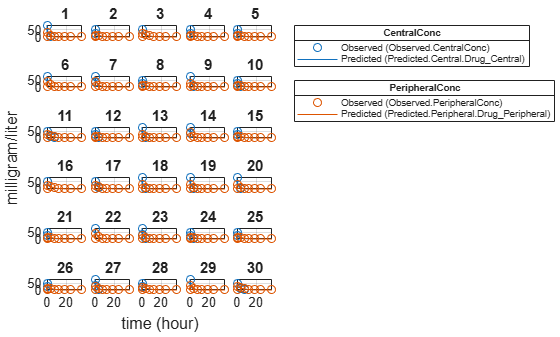For the `Cl_Central` and `Q12` parameters, all males had the same estimates, and similarly for the females. For the `Central` and `Peripheral` parameters, all young individuals had the same estimates, and similarly for the old individuals.

Estimate Population-Wide Parameters

To better compare the results, fit the model to all of the data pooled together, that is, estimate one set of parameters for all individuals by setting the `'Pooled'` name-value pair argument to `true`. The warning message tells you that this option will ignore any category-specific information (if they exist).

`pooledFit = sbiofit(model,gData,responseMap,estimatedParam,dose,'Pooled',true);`
```Warning: CategoryVariableName property of the estimatedInfo object is ignored when using the 'Pooled' option. ```

Plot Results

Plot the fitted results versus the original data. Although a separate plot was generated for each individual, the data was fitted using the same set of parameters (that is, all individuals had the same fitted line).

```t = plot(pooledFit); % Resize the figure. t.hFig.Position(:) = [100 100 1280 800];```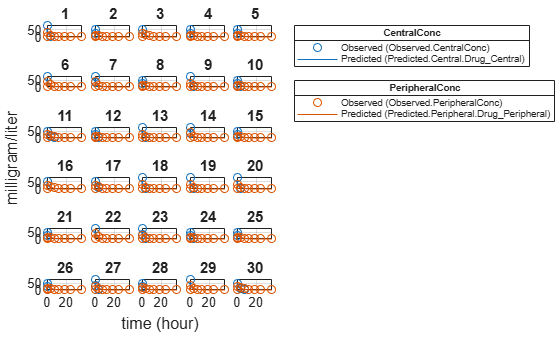Compare Residuals

Compare residuals of `CentralConc` and `PeripheralConc` responses for each fit.

```t = gData.Time; allResid(:,:,1) = pooledFit.R; allResid(:,:,2) = categoryFit.R; allResid(:,:,3) = vertcat(unpooledFit.R); h = figure; responseList = {'CentralConc', 'PeripheralConc'}; for i = 1:2 subplot(2,1,i); oneResid = squeeze(allResid(:,i,:)); plot(t,oneResid,'o'); refline(0,0); % A reference line representing a zero residual title(sprintf('Residuals (%s)', responseList{i})); xlabel('Time'); ylabel('Residuals'); legend({'Pooled','Category-Specific','Unpooled'}); end % Resize the figure. h.Position(:) = [100 100 1280 800];```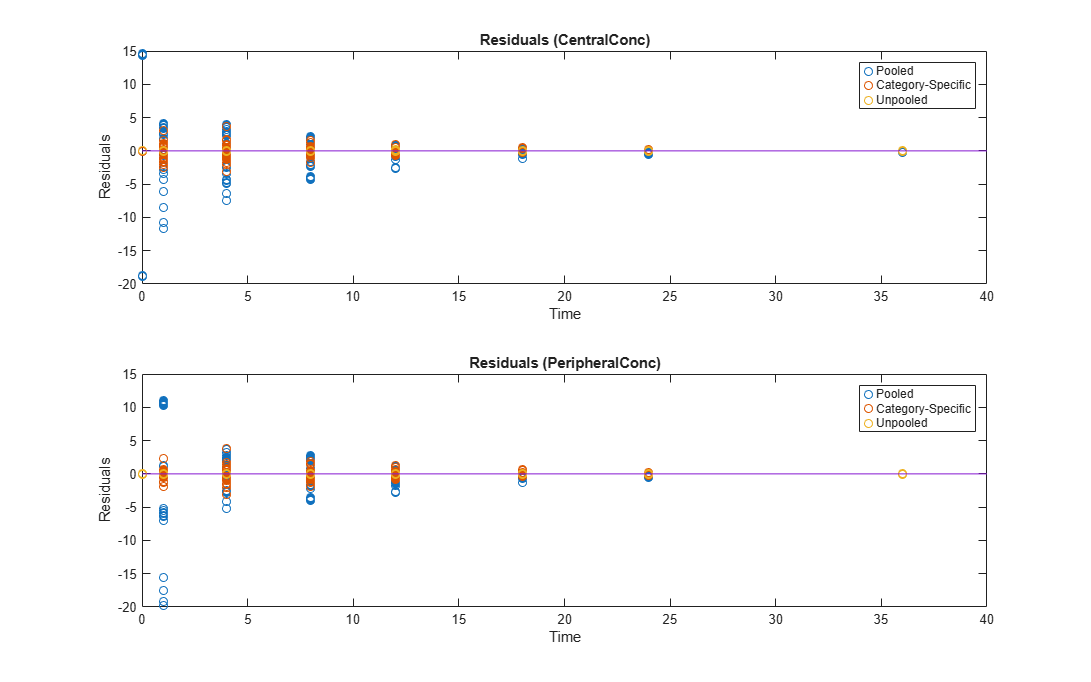As shown in the plot, the unpooled fit produced the best fit to the data as it fit the data to each individual. This was expected since it used the most number of degrees of freedom. The category-fit reduced the number of degrees of freedom by fitting the data to two categories (sex and age). As a result, the residuals were larger than the unpooled fit, but still smaller than the population-fit, which estimated just one set of parameters for all individuals. The category-fit might be a good compromise between the unpooled and pooled fitting provided that any hierarchical model exists within your data.